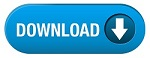Home » SSC » Model Questions for Scientific Assistant (IMD) Exam (Paper-2) PHYSICS – Set 5

a. Speed
b. Velocity
c. Acceleration
d. Free fall

a. Speed
b. Velocity
c. Acceleration
d. Free fall

a. Speed
b. Velocity
c. Acceleration
d. Free fall

a. 5 m/s
b. 10 m/s
c. 15 m/s
d. 20 m/s

a. 1/2 seconds
b. 1 second
c. 2 seconds
d. 4 seconds

#a. increase
b. stop
c. decrease
d. keep the same

a. 30 m/s
b. 35 m/s
c. 40 m/s
d. 20 m/s

a. greater
b. the same
c. double
d. lower

## 9. A falling object has reached a state where the force due to air-resistance balances the force due to gravity. So the object has stopped accelerating called—

a. Inertia
b. Mass
c. Weight
d. Terminal speed

a. Inertia
b. Mass
c. Weight
d. Friction

#